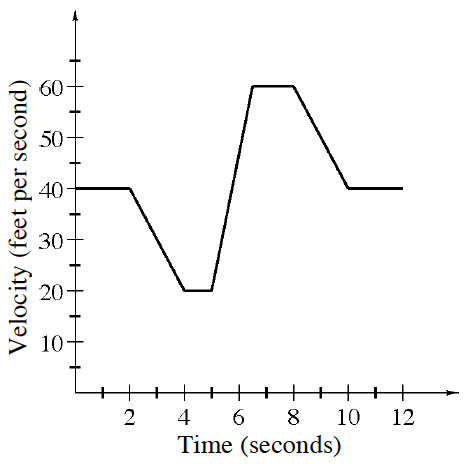### Home > APCALC > Chapter 2 > Lesson 2.2.2 > Problem2-72

2-72.

THE CLUNKER

Tiffani has an old car that she is constantly repairing. One day, she is driving to school when she starts having problems with the car’s fuel injection system. Her velocity (in feet per second) is shown at right.

1. Recall that acceleration is the rate of change of velocity. How is acceleration represented on a velocity graph?

$\text{acceleration }=\text{ rate of change of velocity }= \frac{\frac{\text{feet}}{\text{second}}}{\text{second}}.$

Notice what each axis represents.

2. When is her acceleration negative?

negative acceleration $=$ decreasing velocity. The first time this happens is between $t = 2$ and $t = 4$. When else does this happen?

3. What is her maximum acceleration?

On what interval is Tiffani's velocity increasing the most? Remember, this is a velocity graph.

4. Describe the motion of the vehicle when the acceleration is zero.

$0$ acceleration means that velocity is not changing. It does NOT mean that velocity is $0$. What does it mean then? Notice that Tiffani was moving with $0$ acceleration during three intervals on her journey.

5. How far does Tiffani travel during the $12$ seconds shown in the graph?

Displacement can be determined by calculating the area under a velocity curve.